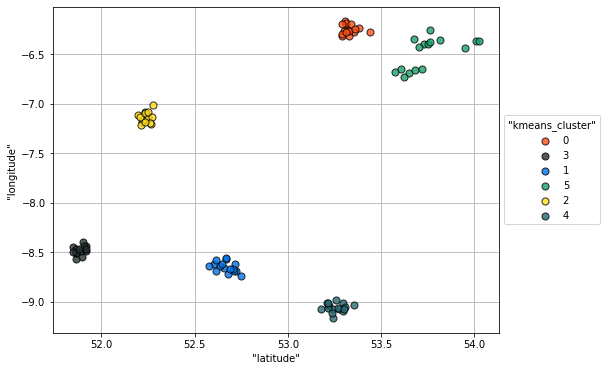### VerticaPy

Python API for Vertica Data Science at Scale

# Clustering¶

Clustering algorithms are used to segment data or to find anomalies. Generally speaking, clustering algorithms are sensitive to unnormalized data, so it's important to properly prepare your data beforehand.

For example, if we consider the 'titanic' dataset, the features 'fare' and 'age' don't have values within the same interval; that is, 'fare' can be much higher than the 'age'. Applying a clustering algorithm to this kind of dataset would create misleading clusters.

To create a clustering model, we'll start by importing the k-means algorithm.

In :
```from verticapy.learn.cluster import KMeans
```

Next, we'll create a model object. Since Vertica has its own model management system, we just need to choose a model name and cluster size.

In :
```model = KMeans("KMeans_sm", n_cluster = 6)
```

We can fit the model to our dataset.

In :
```model.fit("sm_meters", ["latitude", "longitude"])
model.plot()
```
Out:
`<AxesSubplot:xlabel='"latitude"', ylabel='"longitude"'>`While there aren't any real metrics for evaluating unsupervised models, metrics used during computation can help us to understand the quality of the model. For example, a k-means model with fewer clusters and when the k-means score, 'Between-Cluster SS / Total SS' is close to 1.

In :
```model.metrics_
```
Out:value 1201.502 1209.2077 7.7057075 0.993627480208735 ✅
Rows: 1-5 | Columns: 2

This concludes this lesson on clustering models in VerticaPy. We'll look at time series models in the next lesson.